### Chemical Reactions And Equations Class 10th Science And Technology Part 1 MHB Solution Exercise

###### Exercise
Question 1.

Choose the correct option from the bracket and explain the statement giving reason.

(Oxidation, displacement, electrolysis, reduction, zinc, copper, double displacement, decomposition)

a. To prevent rusting, a layer of ........ metal is applied on iron sheets.

b. The conversion of ferrous sulphate to ferric sulphate is ........ reaction.

c. When electric current is passed through acidulated water ........ of water takes place.

d. Addition of an aqueous solution of ZnSO4 to an aqueous solution of BaCl2 is an example of ....... reaction.

a. To prevent rusting, a layer of zinc metal is applied on iron sheets.

Explanation:

Galvanization is a method to prevent rusting.

i. In this method, a thin layer of zinc is applied on iron sheets to prevent rusting/corrosion.

ii. First rusting of zinc takes place (more electropositive than iron).

iii. After few seasons, zinc layer goes away.

iv. Then, iron starts rusting.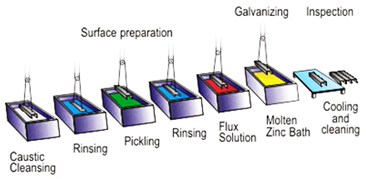b. The conversion of ferrous sulphate to ferric sulphate is oxidation reaction.

Explanation:

Oxidation means losing of electrons.

2FeSO4→ Fe2(SO4)3

Ionic reaction Fe2+ + SO42-→ 2Fe3+ + 3SO42-

As we can observe that in ionic reaction that Fe2+ changes to Fe3+. In this, the positive charge increases by one unit. This means ferrous ion loses one electron. Thus, the above reaction is an oxidation reaction.

c. When electric current is passed through acidulated water electrolysis of water takes place.

Explanation:

On passing electric current through acidulated water (a water contains acid), water decomposes into hydrogen and oxygen gases. This decomposition takes place due to the presence of electrical energy. The reaction takes place is called electrolysis.

Electrolysis: 2H2O(l) → 2H2↑ + O2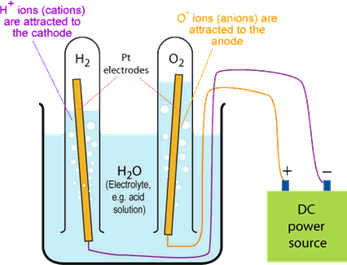d. Addition of an aqueous solution of ZnSO4 to an aqueous solution of BaCl2 is an example of double displacement reaction.

Explanation:

On addition of ZnSO4 and BaCl2, the following reaction takes place:

ZnSO4 + BaCl2→ ZnCl2 + BaSO4

In the reaction, the exchange of ions are taking place. The reaction in which exchange of ions take place to form precipitate are called double displacement reaction.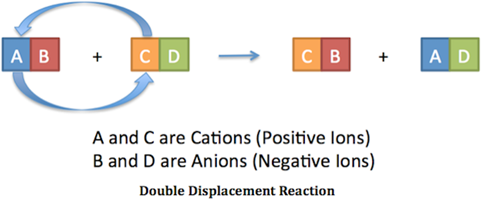Question 2.

What is the reaction called when oxidation and reduction take place simultaneously? Explain with one example.

When oxidation and reduction takes place simultaneously, the reaction is called redox reaction.

Oxidation: losing of electrons Reduction: gaining of electrons

Redox Reaction = Reduction + oxidation

For example:

CuO + H2→ Cu + H2O

i. In the reaction, CuO loses oxygen atom which means that reduction of CuO(copper oxide) takes place.

ii. H2 (hydrogen) takes up oxygen atom.

iii. As a result, formation of water takes place. This means hydrogen undergoes oxidation.

iv. Oxidation and reduction are taking place at the same time, thus it a redox reaction.

Another example: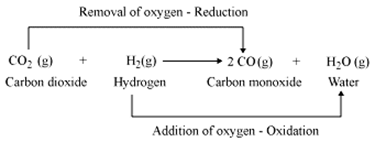Question 3.

How can the rate of the chemical reaction, namely, decomposition of hydrogen peroxide be increased?

Decomposition of hydrogen peroxide is a slow process:

2H2O2(l) → 2H2O + O2↑ (slow reaction)

But we can increase the rate of reaction by using a particular catalyst, i.e., Manganese dioxide powder (MnO2).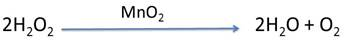A catalyst is a substance which increases the rate of reaction without causing any chemical change.

Question 4.

Explain the term reactant and product giving examples.

Reactants – The substances which take part in a chemical reaction are called reactants.

Products – The substances which are formed due to the formation of new bonds in a chemical reaction are called products.

For example: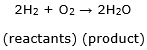Here, H2 and O2 take part in the chemical reaction, hence they are reactants. H2O is formed by the formation of new bonds; hence it is a product.

Question 5.

Explain the types of reaction with reference to oxygen and hydrogen. Illustratre with examples.

Types of reaction with reference to oxygen and hydrogen:

i. Decomposition reaction

2H2O2→ 2H2O + O2

In this reaction hydrogen peroxide undergoes decomposition

to form water and oxygen gas.

ii. Redox reaction

CuO + H2→ Cu + H2O

In this reaction, oxidation and reduction taking place at the

same time.

iii. Electrolysis of water (decomposition)

2H2O(l) → 2H2↑ + O2

In this decomposition of water takes place to release hydrogen and oxygen gas.

Question 6.

Explain the similarity and difference in two events, namely adding NaOH to water and adding CaO to water.

Reaction between NaOH and water: NaOH + H2O → Na+ + OH-

Reaction between CaO and water : CaO + H2O → Ca(OH)2 + heat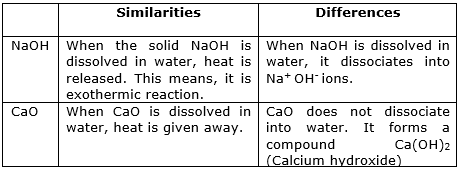Question 7.

Explain the following terms with examples.

Endothermic reaction

Endothermic reaction: In endothermic reaction, heat is either absorbed from the surroundings or heat has to supplied from outside. It means an endothermic reaction needs or takes in heat to continue.

For example: When a solid ammonium chloride (NH4Cl) is dissolved into water, you will observe that the tube becomes colder.

NH4Cl (s) + H2O → NH4Cl (aq) – Heat

In the above reaction, heat is absorbed from surroundings.

Question 8.

Explain the following terms with examples.

Combination reaction

Combination reaction: When two or more reactants combine in a reaction to form a single product, it is called a combination reaction.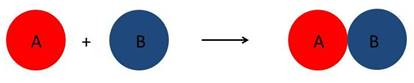For example: A magnesium strip is heated in the presence of air to form a new product, i.e., magnesium oxide (MgO)

Mg + O2→ MgO

In the above reaction, the combination of Mg and O2 leads to the formation of manganese oxide.

Question 9.

Explain the following terms with examples.

Balanced equation

Balanced equation: When the number of atoms of elements in the reactants is equal to the number of atoms of those elements in the products, such a equation is called ‘balanced equation’.

For example: H2 + O2→ 2H2O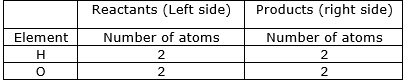In the above table, you can see that number of atoms of reactants are equal to the number of atoms of products. Thus, the reaction is a ‘balanced equation’.

Question 10.

Explain the following terms with examples.

Displacement reaction

Displacement reaction: The reaction in which the ion of a more reactive element displaces the ion of a less reactive metal by forming its own ions. Such reaction is called displacement reaction.

In short, displacement reaction is a reaction in which a more reactive element displaces the less reactive element to form its new compound.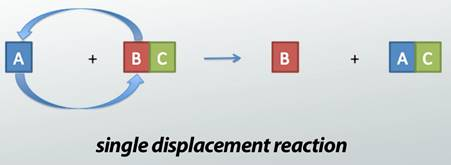For example: CuSO4 + Zn → ZnSO4 + Cu

In the reaction, Zn2+ ions formed from Zn atoms take the place of Cu2+ ions in copper sulphate (zinc is more reactive).

It means that Zn displaces Cu from CuSO4.

Question 11.

Give scientific reasons.

When the gas formed on heating limstone is passed through freshly prepared lime water, the lime water turns milky.

When limewater (CaOH)2 is passed through a gas, CO2, the following reaction takes place: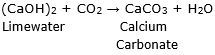⇒ In this reaction, when limewater comes in contact with the gas released in the form of an effervescence, it turns milky. This is chemical test for carbon dioxide gas.

⇒ When limewater turns milky, it is confirmed that the effervescence is of carbon dioxide.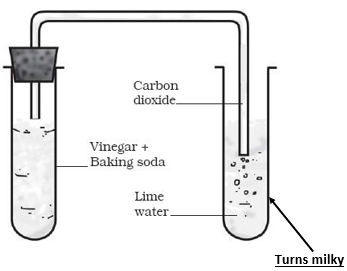⇒ ‘Lime water turning milky’ is the test of carbon dioxide gas.

Question 12.

Give scientific reasons.

It takes time for pieces of Shahabad tile to disappear in HCl, but its powder disappears rapidly.

On addition of dilute HCl in pieces of Shahabad tile, the rate of reaction is very slow. On the other hand, on addition of dilute HCl in powder form of Shahabad tile, the rate of reaction is fast because:

⇒ The rate of reaction depends upon the size of the particles of the reactants.

⇒ Smaller the size of reactant, higher will be its rate of reaction.

⇒ Greater the size of reactant, lesser will be its rate of reaction.

Question 13.

Give scientific reasons.

While preparing dilute sulphuric acid from concentrated sulphuric acid in the laboratory, the concentrated sulphuric acid is added slowly to water with constant stirring.

When concentrated sulphuric acid is diluted with water, a very large amount of heat is released. It is very dangerous and can cause accident.

⇒ To avoid this, a required amount of water is taken in a beaker.

⇒ Small quantity of sulphuric acid is added into the water.

⇒ Constant stirring has to be done to decrease the releasing heat.

⇒ As a result, only a small amount of heat is released.

Note: In chemical labs, it always advised that we should add acid in water, not water in acid.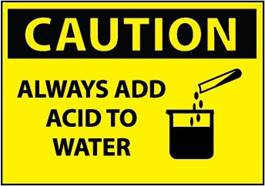Question 14.

Give scientific reasons.

It is reccommended to use air tight container for storing oil for long time.

It is recommended to use air tight container for storing oil for long time because:

⇒ When oil comes in contact with air, it undergoes air oxidation.

⇒ This can make the oil rancid (a foul smell).

⇒ Thus, it is advised to use air tight container for storing oil to prevent it from exposure of air.

Question 15.

Observe the following picture a write down the chemical reaction with explanation.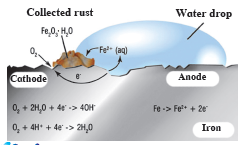⇒ The picture given shows the corrosion od iron.

⇒ The chemical formula of rust is Fe2O3.H2O.

⇒ The rust on iron does not form by a simple reaction of oxygen with iron surface. The rust is formed by an electrochemical reaction.

⇒ Different regions on the surface of iron become anode and cathode.

⇒ Fe is oxidised to Fe2+ (anode)

Fe (s) → Fe2+ (aq) + 2e-

⇒ O2 is reduced to form water (cathode)

O2 (g) + 4H+ (aq) + 4 e- → 2H2O

⇒ When Fe2+ ions migrate from the anode region they react with water and further get oxidised to form Fe3+ ions.

⇒ A redish coloured hydrated oxide is formed from Fe3+ ions.

⇒ It is called rust which collects on the surface.

⇒ Due to various components of atmosphere, oxidation of metals takes place, consequently resulting in their damage. This is called ‘corrosion’.

⇒ Iron rusts and a redish coloured layer is collected on it.

⇒ This is corrosion of iron.

Question 16.

Identify from the following reactions the reactants that undergo oxidation and reduction.

a. Fe + S → FeS

b. 2Ag2O → 4 Ag + O2

c. 2Mg + O2→ 2MgO

d. NiO + H2→ Ni + H2O

a. Fe + S → FeS

In the given reaction, Fe is giving 2 electrons to Sulphur. Sulphur is accepting those electrons. This means:

⇒ Fe undergoes oxidation (to lose electrons)

⇒ Sulphur undergoes reduction (to accept electrons)

b. 2Ag2O → 4 Ag + O2

⇒ Ag in Ag2O has oxidation state= +1

Ag in Ag(s) has oxidation state = 0

This means Ag undergoes reduction

⇒ O in Ag2O has oxidation state = -2

O in O2 has oxidation state = 0

This means oxygen undergoes oxidation

c. 2Mg + O2→ 2MgO

First write the half reactions:

Mg0 → Mg2+ + 2e-

O2 + 4e-→ O2-

⇒ Oxygen goes from 0 to -2. Thus, it undergoes reduction.

⇒ Magnesium goes from 0 to +2. Thus, it undergoes oxidation

d. NiO + H2→ Ni + H2O

First write the half reactions:

2H0 - 2e-→ 2H1

Ni2+ + 2e-→ Ni0

⇒ Hydrogen goes from 0 to 1. Thus, it undergoes oxidation.

⇒ Nickel goes from 2 to 0. Thus, it undergoes reduction.

Question 17.

Balance the following equation stepwise.

H2S2O7(l) + H2O(l) → H2SO4(l)

Balanced equation: H2S2O7 + H2O → 2H2SO4

Explanation:

⇒ Step 1: Write the given unbalance equation

H2S2O7 + H2O(l)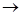H2SO4(l)

⇒ Step 2: Compare the number of atoms of reactants with the number of atoms of products.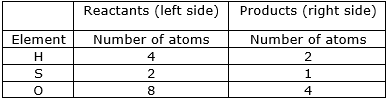⇒ Step 3: Now, if we multiply 2 in the products, we will get the equal number of atoms as reactants.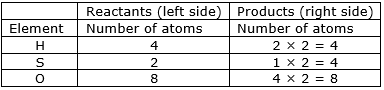⇒ Write down the final balanced equation:

H2S2O7 + H2O → 2H2SO4

Question 18.

Balance the following equation stepwise.

SO2(g) + H2S(aq) → S(s) + H2O (l)

Balanced equation: SO2(g) + 2H2S(aq)3S(s) + 2H2O (l)

Explanation:

⇒ Step 1: Write the given unbalanced equation

SO2(g) + H2S(aq)S(s) + H2O (l)

⇒ Step 2: Compare the number of atoms of reactants with the number of atoms of products.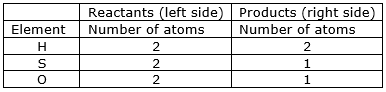⇒ Step 3: Now, let us consider oxygen atom. If we multiply 2 in the product (in H2O), we will get the equal number of atoms as in reactants (SO2)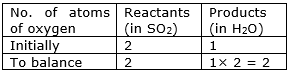⇒ Step 4: Write the resulting equation:

SO2 + H2S + 2H2O

⇒ Step 5: Now check whether the equation is balanced or not by comparing the atoms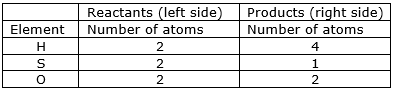We find that the equation is not balanced yet. As the number of

sulphur and hydrogen atoms are unequal on the two sides.

First balance the hydrogen number.

⇒ Step 6: Now, let us consider hydrogen atom. If we multiply 2 in the reactant (in H2S), we will get the equal number of atoms as in product (H2O)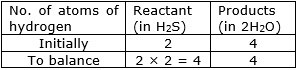⇒ Step 7: Write the resulting equation:

SO2 + 2H2S + 2H2O

⇒ Step 8: Now check whether the equation is balanced or not by comparing the atoms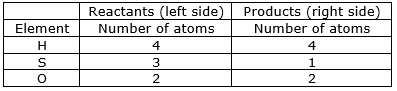We find that the equation is not balanced yet. As the number of

sulphur atom is unequal on the two sides.

⇒ Step 9: Now, let us consider sulphur atom. If we multiply 3 in the product (S), we will get the equal number of atoms as in reactants (SO2 and H2S)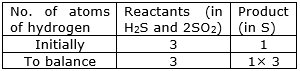⇒ Step 10: Write the resulting equation:

SO2 + 2H23S + 2H2O

⇒ Step 11: Now, compare the atoms of both the sides of all the elements. Write down the final balanced equation:

SO2 + 2H23S + 2H2O

Question 19.

Balance the following equation stepwise.

Ag(s) + HCl(aq) → AgCl + H2

Balanced equation: 2Ag + 2HCl2AgCl + H2

Explanation:

⇒ Step 1: Write the given unbalanced equation

Ag + HClAgCl + H2

⇒ Step 2: Compare the number of atoms of reactants with the number of atoms of products.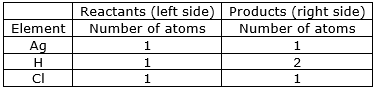⇒ Step 3: Now, First we consider the element having unequal no. of atoms on both sides. Thus, let us consider hydrogen atom. If we multiply 2 in the reactant (in HCl), we will get the equal number of atoms as in product (H2)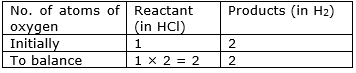⇒ Step 4: Write the resulting equation:

Ag + 2HCl → AgCl + H2

⇒ Step 5: Now check whether the equation is balanced or not by comparing the atoms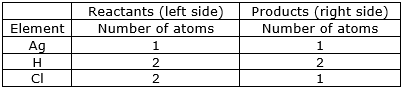We find that the equation is not balanced yet. As the number of

chlorine atom is unequal on the two sides.

First balance the chlorine number.

⇒ Step 6: Now, let us consider chlorine atom. If we multiply 2 in the product (in AgCl), we will get the equal number of atoms as in reactant (in HCl)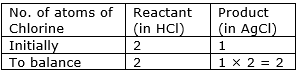⇒ Step 7: Write the resulting equation:

Ag + 2HCl2AgCl + H2

⇒ Step 8: Now check whether the equation is balanced or not by comparing the atoms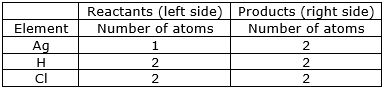We find that the equation is not balanced yet. As the number of

silver atom is unequal on the two sides.

⇒ Step 9: Now, let us consider silver atom. If we multiply 2 in the reactant (Ag), we will get the equal number of atoms as in product (AgCl)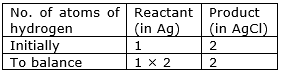⇒ Step 10: Write the resulting equation:

2Ag + 2HCl2AgCl + H2

⇒ Step 11: Now, compare the atoms of both the sides of all the elements. Write down the final balanced equation:

2Ag + 2HCl2AgCl + H2

Question 20.

Balance the following equation stepwise.

NaOH (aq) + H2SO4(aq) → Na2SO4(aq) + H2O(l)

Balanced equation: 2NaOH + H2SO4Na2SO4 + 2H2O

Explanation:

⇒ Step 1: Write the given unbalanced equation

NaOH + H2SO4Na2SO4 + H2O

⇒ Step 2: Compare the number of atoms of reactants with the number of atoms of products.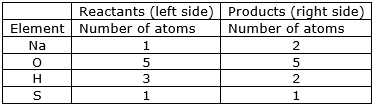⇒ Step 3: Now, first we consider the element having unequal no. of atoms on both sides. Thus, let us consider sodium atom. If we multiply 2 in the reactant (in 2NaOH), we will get the equal number of atoms as in product (Na2SO4)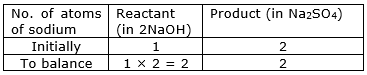⇒ Step 4: Write the resulting equation:

2NaOH + H2SO4Na2SO4 + H2O

⇒ Step 5: Now check whether the equation is balanced or not by comparing the atoms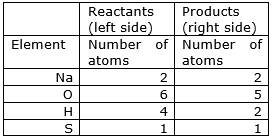We find that the equation is not balanced yet. As the number of Oxygen, hydrogen and sulphur atoms are unequal on the two sides.

First balance the hydrogen number.

⇒ Step 6: Now, let us consider hydrogen atom. If we multiply 2 in the product (in H2O), we will get the equal number of atoms as in reactant (in 2NaOH and H2SO4))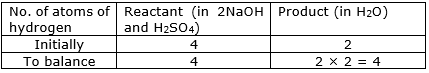⇒ Step 7: Write the resulting equation:

2NaOH + H2SO4Na2SO4 + 2H2O

⇒ Step 8: Now check whether the equation is balanced or not by comparing the atoms.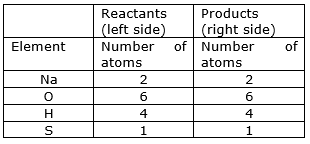We find that the equation is now balanced yet.

⇒ Step 11: Write down the final balanced equation:

2NaOH + H2SO4Na2SO4 + 2H2O

Question 21.

Identify the endothermic and exothermic reaction.

a. HCl + NaOH → NaCl + H2O + heat

b. 2KClO3(s)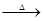2KCl(s) + 3O2

c. CaO + H2O → Ca(OH)2 + heat

d. CaCO3(s)CaO(s) + CO2 ↑

Exothermic reaction: A reaction in which heat is released when reactants changes into products.

Endothermic reaction: A reaction in which heat is either supplied or absorbed from the surroundings.

a. HCl + NaOH → NaCl + H2O + heat

The given reaction is exothermic because in the reaction heat is released when reactants transformed into products.

b. 2KClO3 (s)2KCl(s) + 3O2

The given reaction is an endothermic reaction because in the reaction, heat has been supplied to continue the process.

c. CaO + H2O → Ca(OH)2 + heat

The given reaction is an exothermic reaction because in the reaction heat is released when reactants transformed into products.

d. CaCO3 (s)CaO(s) + CO2

The given reaction is an endothermic reaction because in the reaction, heat has been supplied to continue the process.

Question 22.

Match the column in the following table.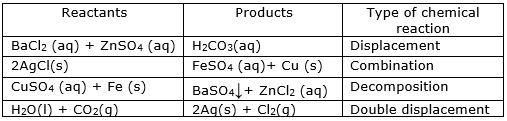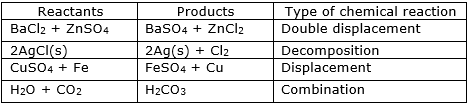Explanation:

⇒ BaCl2 + ZnSO4 → BaSO4 + ZnCl2

The given reaction is an example of double displacement reaction. In this reaction, exchange of ions of Ba and Zn are taking place.

Note: Double displacement reaction is a reaction in which the ions of metals exchange to form different products.

⇒ 2AgCl → 2Ag + Cl2

The given reaction is an example of decomposition reaction. In the given reaction, AgCl decomposes into Ag and Cl2.

Note: Decomposition reaction is a reaction in which only one reactant decomposes into two or more products.

⇒ CuSO4 + Fe → FeSO4 + Cu

The given reaction is an example of displacement reaction. In this reaction, the most reactive metal (Fe) displaces the other metal.

Note: Displacement reaction is a reaction in which the ions of the most reactive metals displaces the ions of the less reactive metal to form its own ions.

⇒ H2O + CO2→ H2CO3

The given reaction is an example of combination reaction. In this reaction, H2O and CO2 combines together to form H2CO3 (carbonic acid).

Note: Combination reaction is a reaction in which two reactants combine together to form a new product.

## PDF FILE TO YOUR EMAIL IMMEDIATELY PURCHASE NOTES & PAPER SOLUTION. @ Rs. 50/- each (GST extra)

SUBJECTS

HINDI ENTIRE PAPER SOLUTION

MARATHI PAPER SOLUTION
SSC MATHS I PAPER SOLUTION
SSC MATHS II PAPER SOLUTION
SSC SCIENCE I PAPER SOLUTION
SSC SCIENCE II PAPER SOLUTION
SSC ENGLISH PAPER SOLUTION
SSC & HSC ENGLISH WRITING SKILL
HSC ACCOUNTS NOTES
HSC OCM NOTES
HSC ECONOMICS NOTES
HSC SECRETARIAL PRACTICE NOTES

2019 Board Paper Solution

HSC ENGLISH SET A 2019 21st February, 2019

HSC ENGLISH SET B 2019 21st February, 2019

HSC ENGLISH SET C 2019 21st February, 2019

HSC ENGLISH SET D 2019 21st February, 2019

SECRETARIAL PRACTICE (S.P) 2019 25th February, 2019

HSC XII PHYSICS 2019 25th February, 2019

CHEMISTRY XII HSC SOLUTION 27th, February, 2019

OCM PAPER SOLUTION 2019 27th, February, 2019

HSC MATHS PAPER SOLUTION COMMERCE, 2nd March, 2019

HSC MATHS PAPER SOLUTION SCIENCE 2nd, March, 2019

SSC ENGLISH STD 10 5TH MARCH, 2019.

HSC XII ACCOUNTS 2019 6th March, 2019

HSC XII BIOLOGY 2019 6TH March, 2019

HSC XII ECONOMICS 9Th March 2019

SSC Maths I March 2019 Solution 10th Standard11th, March, 2019

SSC MATHS II MARCH 2019 SOLUTION 10TH STD.13th March, 2019

SSC SCIENCE I MARCH 2019 SOLUTION 10TH STD. 15th March, 2019.

SSC SCIENCE II MARCH 2019 SOLUTION 10TH STD. 18th March, 2019.

SSC SOCIAL SCIENCE I MARCH 2019 SOLUTION20th March, 2019

SSC SOCIAL SCIENCE II MARCH 2019 SOLUTION, 22nd March, 2019

XII CBSE - BOARD - MARCH - 2019 ENGLISH - QP + SOLUTIONS, 2nd March, 2019

HSC Maharashtra Board Papers 2020

(Std 12th English Medium)

HSC ECONOMICS MARCH 2020

HSC OCM MARCH 2020

HSC ACCOUNTS MARCH 2020

HSC S.P. MARCH 2020

HSC ENGLISH MARCH 2020

HSC HINDI MARCH 2020

HSC MARATHI MARCH 2020

HSC MATHS MARCH 2020

SSC Maharashtra Board Papers 2020

(Std 10th English Medium)

English MARCH 2020

HindI MARCH 2020

Hindi (Composite) MARCH 2020

Marathi MARCH 2020

Mathematics (Paper 1) MARCH 2020

Mathematics (Paper 2) MARCH 2020

Sanskrit MARCH 2020

Important-formula

THANKS